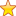# Optimal Page Replacement Algorithm in Python

Data in an operating system is present in the form of chunks of memory called pages. Pages are brought in and out of the main memory as they are demanded by the operating system for processing. Pages which are most frequently used are kept in the primary memory, on the other hand, those that are not used frequently are sent back to the secondary memory.### Program in python

```
#programming code for Optimal Page Replacement Algorithm

#entering the number of frames

print(“Enter the number of frames: ”, end = “”)

storage = int(input())

f, fault, pf = [], 0, ‘No’

#entering the referencing string

print(“Fill the reference string”, end =””)

string = list(map(int, input().strip().split()))

print(“\nString|Frame -> t”, end =’’)

#calculating the number of requests

for i in range (storage)

print(I, end= ‘ ‘)

print(“Fault\n  ↓\n”)

occurrence = [None for i in range(storage)]

#calculating the number of faults

for i in range (len(s));

if s[i] not in f;

if len(f)< storage

f.append(s[i])

else:

for x in range(len(f));

if f[x] not in s[i+1]:

f[x] = s[i]

break

else:

occurrence[x] = s[i+1:].index(f[x])

else:

#calculating the fault rate

if[occurance.index(max(occurence))] = s[i]

fault += 1

pf= ‘Yes’

else:

pf = ‘No’

print(“ %d\t\t” %s[i], end= ‘’)

for x in f:

print (x, end = ‘ ’)

for x in range (storage – len(f)):

print(‘ ’, end = ‘ ’)

for x in range (storage – len(f)):

print (‘ ’, end = ‘ ’)

print (“%s” %pf )

print(“\n Total number of requests: %d\n Total number of page faults: %d, Fault Rate: %0.2f%%"%(len(s),fault,(fault/len(s))*100)”))
```

### Output

```Enter the number of frames:

3

Fill the reference string:

3 2 1 5 3 9

Total number of requests:

6

Total number of page faults:

2

Fault rate:

2```
Summary
Review Date
Reviewed Item
optimal page replacement algorithm in python
Author Rating
5